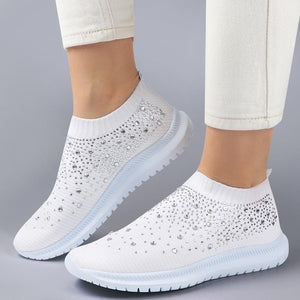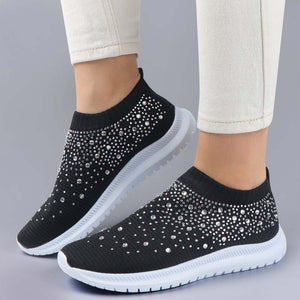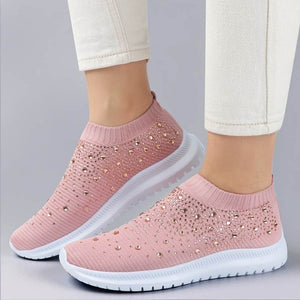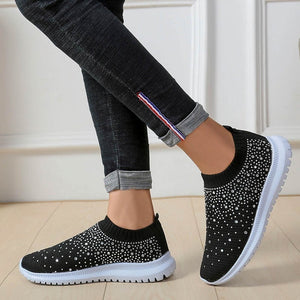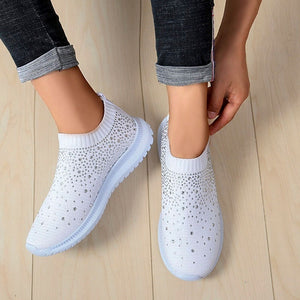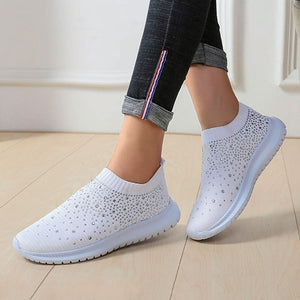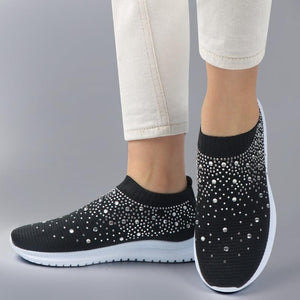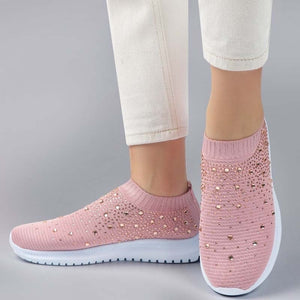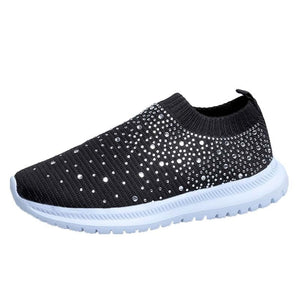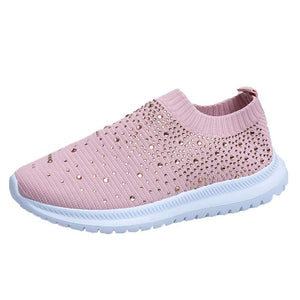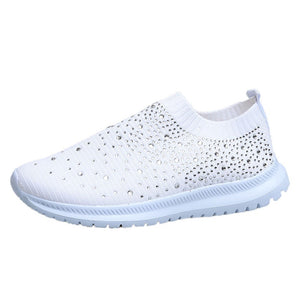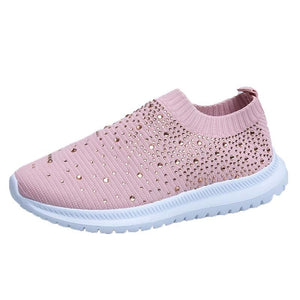# Women Crystals Sneakers Comfy Sport Running Shoes Sparkly Trainers Breathable Mesh Tenis Feminino

Regular price
\$5.36
Sale price
\$5.36
Regular price
\$150.00
Sold out
Unit price
per

Color:White;Black;Pink
Size:35-43
EUR 34 = US 4 = UK 2 = 22 CM = 8.66 INCH
EUR 35 = US 5 = UK 3 =22.5 CM = 8.86 INCH
EUR 36 = US 5.5 = UK 3.5 = 23 CM = 9.06 INCH
EUR 37 = US 6 = UK 4 = 23.5 CM = 9.25 INCH
EUR 38 = US 6.5 = UK 4.5 = 24 CM = 9.45 INCH
EUR 39 = US 7.5 = UK 5 = 24.5 CM =9.65 INCH
EUR 40 = US 8.5 = UK 5.5 = 25 CM =9.84 INCH
EUR 41 = US 9 = UK 6 = 25.5 CM =10.04 INCH
EUR 42 = US 10 = UK 6.5 = 26 CM =10.24 INCH
EUR 43 = US 10.5 = UK 7 = 26.5 CM = 10.43 INCH